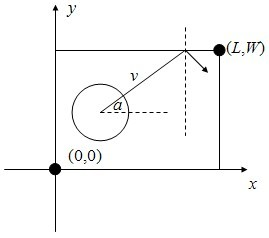﻿ N-B-U-T Online Judge ::  台球碰撞
• ###  台球碰撞

• 时间限制: 1000 ms　内存限制: 65535 K
• 问题描述
• 在平面直角坐标系下，台球桌是一个左下角在(0,0)，右上角在(L,W)的矩形。有一个球心在(x,y)，半径为R的圆形母球放在台球桌上（整个球都在台球桌内）。受撞击后，球沿极角为a的射线（即：x正半轴逆时针旋转到此射线的角度为a）飞出，每次碰到球桌时均发生完全弹性碰撞（球的速率不变，反射角等于入射角）。如果球的速率为vs个时间单位之后球心在什么地方？

• 输入
• 输入文件最多包含25组测试数据，每个数据仅一行，包含8个正整数L,W,x,y,R,a,v,s（100<=L,W<=105, 1<=R<=5, R<=x<=L-R, R<=y<=W-R, 0<=a<360, 1<=v,s<=105），含义见题目描述。L=W=x=y=R=a=v=s=0表示输入结束，你的程序不应当处理这一行。
• 输出
• 对于每组数据，输出仅一行，包含两个实数x, y，表明球心坐标为(x,y)。x和y应四舍五入保留两位小数。
• 样例输入
• ```100 100 80 10 5 90 2 23
110 100 70 10 5 180 1 9999
0 0 0 0 0 0 0 0
```
• 样例输出
• ```80.00 56.00
71.00 10.00
```
• 提示
• `无`
• 来源
• `湖南省第六届大学生计算机程序设计竞赛`
• 操作
﻿